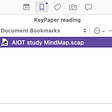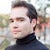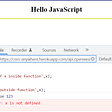# CoDEVIANT #1 (3/21/19)

• A: Not smart
• B: Being an opera singer is a kind of a full time deal as well.
• C: This is just a lick to me, cuh; if I learned I had the power to turn rain into titanium or gold, I’d be outta here. Sorry, not sorry.

# NOTE: this is indexed from [1..n] (not zero indexed!)

1. We need to make a collection of things to look out for. Our vowels! a, e, i, o, u, y.
2. We need a new array that starts out as empty to hold our answer.
3. We need to turn the strings we receive as arguments to the function into arrays.
4. Then we need to find where the matches are between the elements in our vowelArray and our strings-converted-into-arraysArrays.
5. When there are matches, push them into an answerArray.
6. Return the answer array. Profit.
`let vowels = [‘a’,’A’,’e’,’E’,’i’,’I’,’o’,’O’,’u’,’U’,’y’, ‘Y’];`
`let answer = [];`
`let wordArray = word.split(‘’);`
`wordArray.forEach((letter)=>{//fun stuff coming soon!}`
`wordArray.forEach((letter)=>{  vowels.forEach((target) => {  //fun stuff on the way  }}`
`wordArray.forEach((letter)=>{ vowels.forEach((target) => {    if(letter == target) {    answer.push(wordArray.indexOf(letter) + 1);    }  }}`
`function vowelIndices(word){let vowels = [‘a’,’e’,’i’,’o’,’u’,’y’];let answer = [];let wordArray = word.split(‘’);console.log(wordArray);        wordArray.forEach((letter) => {          vowels.forEach((target) => {             if(letter == target) {             answer.push(wordArray.indexOf(letter) + 1);            }          })       })return answer;}`
`let number = 0;let goodNumber = 0;`
`answer.push(goodNumber);`
`function vowelIndices(word){let vowels = [‘a’,’A’,’e’,’E’,’i’,’I’,’o’,’O’,’u’,’U’,’y’, ‘Y’];let answer = [];let number=0;let goodNumber=0;let wordArray = word.split(‘’);console.log(wordArray);wordArray.forEach((letter) => {    number ++;    vowels.forEach((target) => {         if(letter == target) {         goodNumber = number;         answer.push(goodNumber );         }     })})return answer;}`
`function vowelIndices(word) {var arr = [];for(var i = 0; i < word.length; i++) {   if(/[aeioyu]/i.test(word[i])) {  arr.push(i+1);   }}return arr;}`
`return ‘pig’; //returns ‘p’`

# You can assume the array will always be valid, and there will always be one correct answer.

1. Set up a chan of if else statements that look at the current number and compare it to the next number in the sequence
2. If the next number in the sequence is larger, return ‘yes, ascending’
3. If the next number in the sequence is smaller, return ‘yes, descending’
4. If not, then return ‘no’.
`for(let i = 0; i < array.length; i++){currentNumber = array[i];}`
`if(currentNumber < array[i + 1] ) {return ‘yes, ascending’;} else if (currentNumber > array[i+1]){return ‘yes, descending’;} else {return ‘no’;}`
`function isSortedAndHow(array) {let currentNumber;for(let i = 0; i < array.length; i++){  currentNumber = array[i];  if(currentNumber < array[i+1]) {  return ‘yes, ascending’  } else if(currentNumber > array[i+1]) {  return ‘yes, descending’  } else {  return ‘no’;  } }}`
`function isSortedAndHow(arr) {return arr.every((x,i)=>i==0||arr[i]>=arr[i-1])?’yes, ascending’:arr.every((x,i)=>i==0||arr[i]<=arr[i-1])?’yes, descending’:’no’}`

## arr.every( callback[, thisArg])

1. Element = the element of the array currently being processed in the array
2. Index = the index (or address) of the element being processed in the array
3. Array = the array that every was called upon.
`i==0 || arr[i] >= arr[i — 1]`
`aBitOfKnowledgeToEvaluate ? “If it is true” : “If it is false”`
`aBitOfKnowledgeToEvaluate ? “If it’s true”:Somethingelse ? “if the next thing is true”:”it was false”;`
`function isSortedAndHow(arr) {return arr.every((x,i)=>i==0||arr[i]>=arr[i-1])?’yes, ascending’:arr.every((x,i)=>i==0||arr[i]<=arr[i-1])?’yes, descending’:’no’}`

--

--

--

is a web developer, opera singer, actor, and lover of cats. (adrian-rosales.tech)

Love podcasts or audiobooks? Learn on the go with our new app.

## CQI365 Tools: Scrivener BookMark## How to use Redis-Template in Java Spring Boot?## Amazing apps :- https://youtu.be/KqB3m8WFsaY## Internship Experience at an Ed-Tech Startup## Chrono Adventures NFT Dev update 15## 6 Rs in your Journey To Cloud and why to rearchitect## Writing fast, deterministic and accurate Android Integration testsis a web developer, opera singer, actor, and lover of cats. (adrian-rosales.tech)

## Let v/s Var in JavaScript## Basic things to know about “Application Framework”## How to Keep your Custom CSS Styling While Using Bulma.io Components